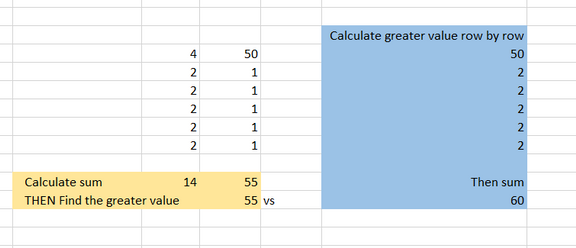# Data Aggregation issue in the Beast Mode Calculation while using Sub-Total and Header Row.

While we use the CASE statements the Aggregation in DOMO first performs SUM and then looks at the condition inside the CASE statement. This means that the following two conditions will provide similar results on the row level, but when the data is aggregated the 2nd condition will only look at the bigger value post SUM() and shall provide incorrect results.

1.

SUM(CASE
WHEN Col1>0 AND (Col1>Col2) THEN Col1
ELSE Col2
END)

2.

(CASE
WHEN SUM(Col1)>0 AND (SUM(Col1)>SUM(Col2)) THEN SUM(Col1)
ELSE SUM(Col2)
END)

Detailed Explanation:

Let's suppose that we look at the first row with two columns, Val1 and Val2. Val1 has 4, Val2 has 50. In this case, Val2 is bigger, and so Val2 will be supplied for that row. So on and so forth. Let's suppose that for every row after that, Val1 is 2, and Val2 is 1, so we end up with a set of rows with Val2 on the first row, and Val1 on all other rows. Then let's sum up all of the values for each row. Let's say we had 5 rows. So we would end up with 50, 2, 2, 2, 2. The sum is then equal to 58.

Cool, so now let's suppose that INSTEAD, we Sum up everything and THEN we apply the beast mode calculation. This means that we sum up everything in Val1, which will be 4 + 2 + 2 + 2 + 2 = 12. Then we sum up Val2 which will be 50 + 1 + 1 + 1 + 1 = 54. Now we do the beast mode. Is Val1 or Val2 bigger? Val2 is, so we will return that, so the display 54. Notice that this sum is 54, instead of 58, which was our sum result with the previous method.

Conclusion:

Always wrap your beast mode in a SUM(CASE STATEMENT) to get the correct value at both row and aggregate levels.

Tagged:

• @NFSharma  i like what you tried to accomplish here.

small feedback to improve engagement.  when possible make it visual. when I was learning about the difference between aggregating row by row versus aggregating on the total column, I found it super useful to do a spreadsheetI applaud you taking the time to writeup your explanation b/c so many people get it wrong / don't understand the difference.

I'd extend your instruction to say careful consideration of whether to calculate CASE before or after aggregation matters in all contexts not just with Subtotal and Total calcs.

THAT SAID.  I do disagree with the generalization in your conclusion.

"Always wrap your beast mode in a SUM(CASE STATEMENT) to get the correct value at both row and aggregate levels."

In general, I agree, usually, yes you'll do math row, by row.  But in certain contexts, you'll want to apply the CASE after aggregation, for example if you were deciding which tax bracket to apply (sorry taxes are on my mind), then you'd apply tax rate AFTER aggregation not before.

OR if you're deciding which HTML formatting to apply in a summary number

OR in market research, "if the count of respondents is below a certain amount then ...  else ..."

Also, from a performance perspective, while the exact query plan and optimization methods of Adrenaline are unknown to us, theoretically, calculating SUM() of 2 columns (especially in a columnar database) and then applying a CASE should execute faster than calculating CASE row by row and then applying a SUM() ... therefore, if both variants would return the same result, the former option should be preferred b/c in most database engines it's likely to be more performant.

This discussion has been closed.